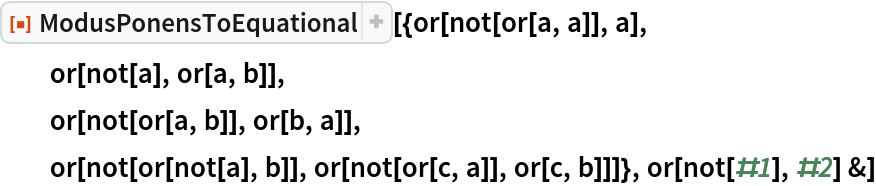Function Repository Resource:

# ModusPonensToEquational

Convert an axiom system from modus ponens to equational form

Contributed by: Matthew Szudzik & Stephen Wolfram
 ResourceFunction["ModusPonensToEquational"][axioms,implies] converts the specified list of modus ponens axioms to equational form, taking implies to be the representation of implication.

## Details and Options

ModusPonensToEquational removes implicit use of modus ponens {x, xy}y as a rule of inference. It generates pure equational axioms that rely only on substitution.

## Examples

### Basic Examples (2)

Convert the Lukasiewicz modus ponens axiom system to equational form:

 In:=Out=Convert to the Hilbert–Ackermann axiom system for propositional logic, giving a function for implies:

 In:=Out=## Requirements

Wolfram Language 11.3 (March 2018) or above

## Version History

• 1.0.0 – 29 November 2018